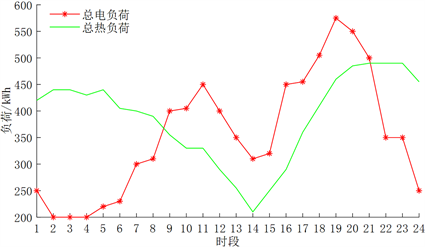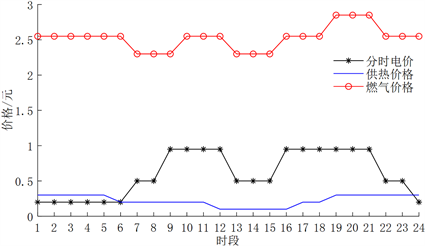# 热电联供系统综合优化调度Comprehensive Optimal Dispatch of Combined Heat and Power System

• 全文下载: PDF(712KB)    PP.532-537   DOI: 10.12677/SG.2018.86058
• 下载量: 389  浏览量: 585

The optimal scheduling of multi-energy systems with natural gas, geothermal and other clean en-ergy sources can effectively improve energy efficiency and reduce environmental pollution. Based on the analysis of the distributed energy supply characteristic and comprehensive consideration of economy, environmental protection and safety, a multi-energy system dispatch mathematical model is established to maximize the comprehensive benefit with the constraint such as the bal-ance of power and heat, the limit of distributed generation and the tie-line power. The three scheduling strategies are guided by electricity price, gas price and coordinated price. The result shows that the integrated optimization dispatch model is superior to the single-objective optimal dispatch model, and the scheme takes into consideration various indexes and achieves the overall optimal effect. The dispatch strategy guided by electricity price and cooperative price is better than that of gas price.

1. 引言

2. 热电联供系统综合优化调度数学模型

2.1. 分布式能源的出力数学模型

2.1.1. CHP系统数学模型

CHP系统包括微型燃气轮机和余热锅炉，微型燃气轮机利用天然气发电，余热锅炉利用微型燃气轮机发电后排气的余热量，提供制热量。CHP系统的数学模式如式(1)~(3)。

${Q}_{MT}\left(t\right)={P}_{MT}\left(t\right)\left(1-{\eta }_{CHP}-{\eta }_{1}\right)/{\eta }_{CHP}$ (1)

${Q}_{CHP.hot}\left(t\right)={Q}_{MT}\left(t\right)×{\eta }_{rec}×CO{P}_{CHP.hot}$ (2)

${\eta }_{rec}=\frac{{T}_{1}-{T}_{2}}{{T}_{1}-{T}_{0}}$ (3)

2.1.2. 燃气锅炉数学模型

${Q}_{GB}\left(t\right)={P}_{GB}\left(t\right)×{\eta }_{GB}$ (4)

2.1.3. 地源热泵数学模型

${Q}_{HP.hot}\left(t\right)=CO{P}_{HP.hot}×{P}_{HP}\left(t\right)$ (5)

2.2. 热电联供系统综合优化调度模型的建立

$\mathrm{min}F=-{\alpha }_{1}{F}_{1}+{\alpha }_{2}{F}_{2}+{\alpha }_{3}{F}_{3}$ (6)

${F}_{1}=\underset{t=1}{\overset{24}{\sum }}\left[{C}_{1}\left(t\right)-{C}_{2}\left(t\right)\right]=\underset{t=1}{\overset{24}{\sum }}\left\{\left[{C}_{e}\left(t\right)×{L}_{e}\left(t\right)+{C}_{h}×{L}_{h}\left(t\right)\right]-\left[\frac{{P}_{gas}}{LH{V}_{ng}}×{C}_{n}+{P}_{grid}\left(t\right)×{C}_{grid}\right]\right\}$ (7)

${F}_{2}=\underset{t=1}{\overset{24}{\sum }}{C}_{3}\left(t\right)=\underset{t=1}{\overset{24}{\sum }}\left[{P}_{CHP}\left(t\right)×\sum {\lambda }_{CHP.i}+{Q}_{GB}\left(t\right)×\sum {\lambda }_{GB.i}+{Q}_{HP}\left(t\right)×\sum {\lambda }_{HP.i}+{P}_{grid}×\sum {\lambda }_{grid.i}\right]$ (8)

${F}_{3}=\underset{t=1}{\overset{24}{\sum }}{C}_{4}\left(t\right)=\underset{t=1}{\overset{24}{\sum }}{P}_{gas}\left(t\right)+{P}_{grid}\left(t\right)$ (9)

${P}_{gas}\left(t\right)=\frac{{P}_{MT}\left(t\right)}{{\eta }_{CHP}}+{P}_{GB}\left(t\right)$ (10)

s.t.

${Q}_{CHP.hot}\left(t\right)+{Q}_{GB}\left(t\right)+{Q}_{HP.hot}\left(t\right)={L}_{h}\left(t\right)$ (11)

${P}_{MT}\left(t\right)+{P}_{grid}\left(t\right)\ge {P}_{HP}\left(t\right)$ (12)

$0\le {P}_{grid}\left(t\right)\le {P}_{grid.\mathrm{max}}$ (13)

$0\le {P}_{CHP}\left(t\right)\le {P}_{CHP.\mathrm{max}}$ (14)

$0\le {Q}_{i}\left(t\right)\le {Q}_{i.\mathrm{max}}$ (15)

3. 热电联供系统综合优化调度策略

3.1. 电价引导(策略1)

1) 当分布式能源站离网运行时：电价高峰时段CHP按其出力上限发电，满足能源站自身用电和部分电负荷；电价低谷时段CHP发电量只用来满足自身用电；电价平时段CHP参与调度；

2) 当分布式能源站并网运行时：电价高峰时段CHP按其出力上限发电，满足能源站自身用电和部分电负荷；电价低谷时段通过CHP发电和大电网买电只满足能源站自身用电；电价平时段CHP参与调度。

3.2. 燃气价格引导(策略2)

1) 当分布式能源站离网运行时：燃气价格高峰时段CHP发电量只用来满足能源站自身用电；燃气价格低谷时段CHP按其出力上限发电；燃气价格平时段CHP参与调度；

2) 当分布式能源站并网运行时：燃气价格高峰时段CHP按其出力下限发电，通过大电网买电来满足能源站自身用电；燃气价格低谷时段CHP按其出力上限发电；燃气价格平时段CHP参与调度。

3.3. 电价与燃气价格协同引导(策略3)

1) 当分布式能源站离网运行时：电价高峰且燃气价格非高峰时段CHP按其出力上限发电，满足能源站自身用电和部分电负荷；电价低谷且燃气价格非低谷时段CHP发电量只用来满足自身用电；电价平时段CHP参与调度；

2) 当分布式能源站并网运行时：电价高峰且燃气价格非高峰时段CHP按其出力上限发电，满足能源站自身用电和部分电负荷；电价低谷且燃气价格非低谷时段通过CHP发电和大电网买电只满足能源站自身用电；电价平时段CHP参与调度。

4. 算例分析Table 1. Parameters of distributed energy resourceTable 2. Equipment pollutant emission factor and environmental valueFigure 1. Daily heating and electrical loadFigure 2. Time-of-use energy priceTable 3. The comparisons between the optimal projects of different scheduling strategy

5. 结论

  曾鸣, 杨雍琦, 刘敦楠, 等. 能源互联网“源–网–荷–储”协调优化运营模式及关键技术[J]. 电网技术, 2016, 40(01): 114-124.  Jin, H., Hong, H., Wang, B., et al. (2005) A New Principle of Synthetic Cascade Utilization of Chemical Energy and Physical Energy. Science in China Series E: Technological Sciences, 48, 163-179.  徐建中. 分布式供电和冷热电联产的前景[J]. 节能与环保, 2002, 10(3): 10-14.  吴利辉, 杨洪海, 吴植华. 三联供系统配置及运行策略的多目标优化分析[J]. 建筑热能通风调, 2012, 31(1): 16-18.  施锦月, 许健, 曾博, 等. 基于热电比可调模式的区域综合能源系统双层优化运行[J]. 电网技术, 2016, 40(10): 2959-2966.  赵峰, 张承慧, 孙波, 等. 冷热电联供系统的三级协同整体优化设计方法[J]. 中国电机工程学报, 2015, 35(15): 3785-3793.  于波, 吴亮, 卢欣, 等. 区域综合能源系统优化调度方法[J]. 电力建设, 2016, 37(1): 70-76.  刘星月, 吴红斌. 太阳能综合利用的冷热电联供系统控制策略和运行优化[J]. 电力系统自动化, 2015, 39(12): 1-6.  段绍辉, 汪伟, 刘中胜, 等. 含光伏的冷热电联供微网系统优化调度方案[J]. 电力系统及其自动化学报, 2013, 25(4): 150-155.  周任军, 冉晓洪, 毛发龙, 等. 分布式冷热电三联供系统节能协调优化调度[J]. 电网技术, 2012, 36( 6): 8-14.  刘洪, 陈星屹, 李吉峰, 等. 基于改进CPSO算法的区域电热综合能源系统经济调度[J]. 电力自动化设备, 2017, 37(6): 193-200.  王艳松, 宋阳阳, 吴昊, 等. 基于禁忌搜索算法的微电网源/荷安全经济调度[J]. 电力系统保护与控制, 2017, 45(20): 21-27.